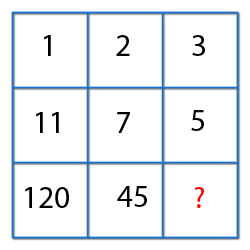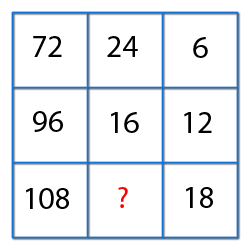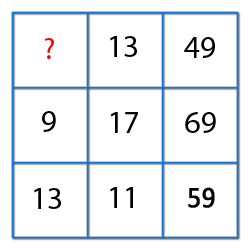﻿ All awareness for Bank PO | Clerk | Railway | IBPS PO | IBPS Clerk | SSC ﻿

### Reasoning

ISBT Reasoning for all banking PO,Clerk,IBPS PO,Railway,SSC,IAS,OAS Exams

Previous12Next

##### Find the missing numbers.1) 18 2) 20 3) 22 4) 24 5) None of these
Explanation :
For First Circle:
Left segment element x right segment Element - 3 = Lower number
3 x 7 - 3 = 18.
For Second Circle:
Left segment element x right segment Element - 4 = Lower number
8 x 2 - 4 = 16.

For Third Circle:
Left segment element x right segment Element - 5 = Lower number
or x = 9 x 3 -5
or x = 22.
Missing number = 22.View Answer
##### Find the missing numbers.1) 12 2) 16 3) 18 4) 20 5) None of these
Explanation : First column : 112 - 12 = 120.
Second Column : 72 - 22 = 45.
Third column : 52 - 32 = 16View Answer
##### Find the missing numbers.1) 10 2) 12 3) 14 4) 18 5) None of these
Explanation : Logic (Row-wise):
(First Element / Second element) x 2 = Third Element.
So, (108/x) x 2 = 18
or x = 12.
Missing Number = 12.View Answer
##### Find the missing number.1) 4 2) 5 3) 8 4) 12 5) None of these
Explanation : Second Row, 2 x 9 + 3 x 17 = 69.

Third Row, 2 x 13 + 3 x 11 = 59.

Let , missing number be x,
So, 2x + 3 x 13 = 49.

or, x = 5.View Answer
##### What should be the missing number to make a complete sequence.1) 8 2) 10 3) 12 4) 16 5) None of these
Explanation : The sequence is : (3+6) +3=12, (5+10) +5 =20
So, (4 +8) = 12View Answer
##### Insert the missing number, to make a complete the sequence.1) 24 2) 30 3) 33 4) 35 5) 39
Explanation : The sequence is:
(3x2) - 1 =5 , (5x2) -2 =8 ,(8x2) -3 =13 , (13x2) - 4 = 22
So,(22x2) -5 =39.View Answer
##### Direction : Select the missing number from the given alternatives.   6        3         8 9       11        ?   7        5        15 96      48      144
 1) 3 2) 4 3) 8 4) 12 5) None of these
Explanation : The sequence is:
6 x (7+9) =96 ;  6 x (11 +3) = 48
So, 8 x (15+3) = 144View Answer
##### Select the missing number from the given responses:1) 2 2) 3 3) 4 4) 5 5) 6
Explanation : The sequence is:  (1 +4+2)2= 49 ; (4+2+2)2 =64
So,(5+3+5)2=169View Answer
##### Which one will replace the question mark ?1) 10 2) 12 3) 16 4) 20 5) None of theseView Answer
##### Which one will replace the question mark ?View Answer